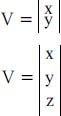NextPrevious

# What do columns and rows mean when discussing vectors?

Vectors can be described by columns and rows. For example, two-dimensional and three-dimensional vectors are usually represented as a single vertical column of numeric values. The following lists such columns in two and three dimensions:A row vector is usually used for problem solving in which a vector is described as V = (x, y, z) during the specification of a problem. But note: Row vectors should not really be used with any mathematical descriptions.

Close

This is a web preview of the "The Handy Math Answer Book" app. Many features only work on your mobile device. If you like what you see, we hope you will consider buying. Get the App